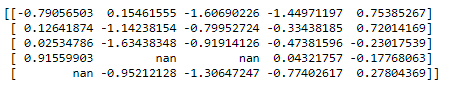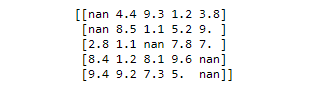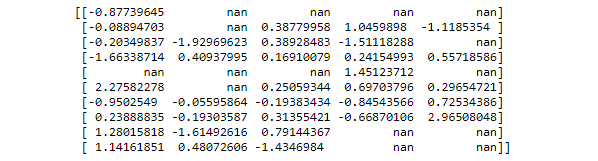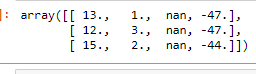# How to randomly insert NaN in a matrix with NumPy in Python ?

• Last Updated : 21 May, 2021

Prerequisites: Numpy

In this article, let’s see how to generate a Python Script that randomly inserts Nan into a matrix using Numpy. Given below are 3 methods to do the same:

Attention geek! Strengthen your foundations with the Python Programming Foundation Course and learn the basics.

To begin with, your interview preparations Enhance your Data Structures concepts with the Python DS Course. And to begin with your Machine Learning Journey, join the Machine Learning - Basic Level Course

### Method 1: Using ravel() function

ravel() function returns contiguous flattened array(1D array with all the input-array elements and with the same type as it). A copy is made only if needed.
Syntax :

`numpy.ravel(array, order = 'C')`

Approach:

• Import module
• Create data
• Choose random indices to Nan value to.
• Pass these indices to ravel() function
• Print data

Example 1:

## Python3

 `import` `numpy as np``import` `pandas as pd` `# number of nan we want to add It will insert 3 nan values to the data.....``n ``=` `3` `# creating dataset``data ``=` `np.random.randn(``5``, ``5``)` `# choosing random indexes to put NaN``index_nan ``=` `np.random.choice(data.size, n, replace``=``False``)` `# adding nan to the data.``data.ravel()[index_nan] ``=` `np.nan``print``(data)`

Output:Example 2: Adding nan to but using randint function to create data. For using np.nan in randint function we must first convert the data into float as np.nan is of float type.

## Python3

 `import` `numpy as np``# number of nan we want to add It will insert 3 nan values to the data.....``n_b ``=` `5` `# creating dataset``data_b ``=` `np.random.randint(``10``, ``100``, size``=``(``5``, ``5``))` `# converting the data to float as nan is also of type float``data_b ``=` `data_b``*``0.1` `# choosing random indexes to put NaN``index_b ``=` `np.random.choice(data_b.size, n_b, replace``=``False``)` `# adding nan to the data.``data_b.ravel()[index_b] ``=` `np.nan``print``(data_b)`

Output:Creating a mask of boolean and applying that mask to the dataset can be one approach to produce the required result.

Approach:

• Import module
• Create data
• Shuffle the mask to randomly apply Nan values
• Apply the mask to the data
• Print data

Example :

## Python3

 `import` `numpy as np` `# creating dataset``X ``=` `10``Y ``=` `5``N ``=` `15` `data ``=` `np.random.randn(X, Y)` `# making a array randomly of same size as data of bool type``mask ``=` `np.zeros(X``*``Y, dtype``=``bool``)` `# marking first n indexes as true``mask[:N] ``=` `True` `# shuffling the mask``np.random.shuffle(mask)``mask ``=` `mask.reshape(X, Y)` `# applying mask to the data``data[mask] ``=` `np.nan``print``(data)`

Output:### Method 3: Using insert()

Using insert() function will convert a whole row or a whole column to NaN. This function inserts values along the mentioned axis before the given indices.
Syntax :

`numpy.insert(array, object, values, axis = None)`

Approach:

• Import module
• Create data
• Use insert Nan values
• Print data

Example:

## Python3

 `import` `numpy as np` `a ``=` `np.array([(``13.0``, ``1.0``, ``-``47.0``), (``12.0``, ``3.0``, ``-``47.0``), (``15.0``, ``2.0``, ``-``44.0``)])` `# adding nan values to the row``np.insert(a, ``2``, np.nan, axis``=``0``)` `# adding nan values to the row``np.insert(a, ``2``, np.nan, axis``=``1``)`

Output:My Personal Notes arrow_drop_up